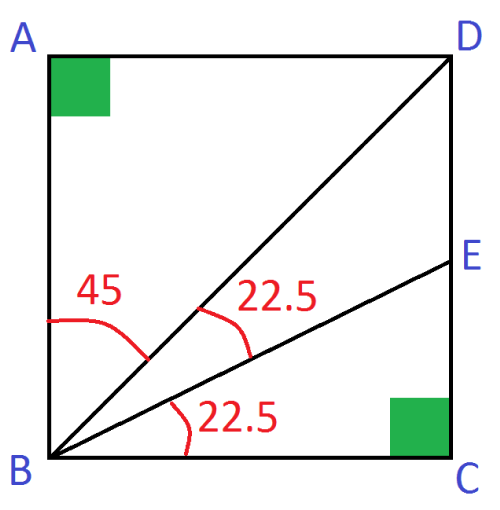# Challenging Geometry Reasoning

Geometry Level 2In the diagram above, $\overline{ABCD}$ is a square. $\overline{BD}$ is the angle bisector of $\angle ABC$ and $\overline{BE}$ is the angle bisector of $\angle DBC$.

Find $\overline{EC}$ in terms of $\overline{DE}$.

×